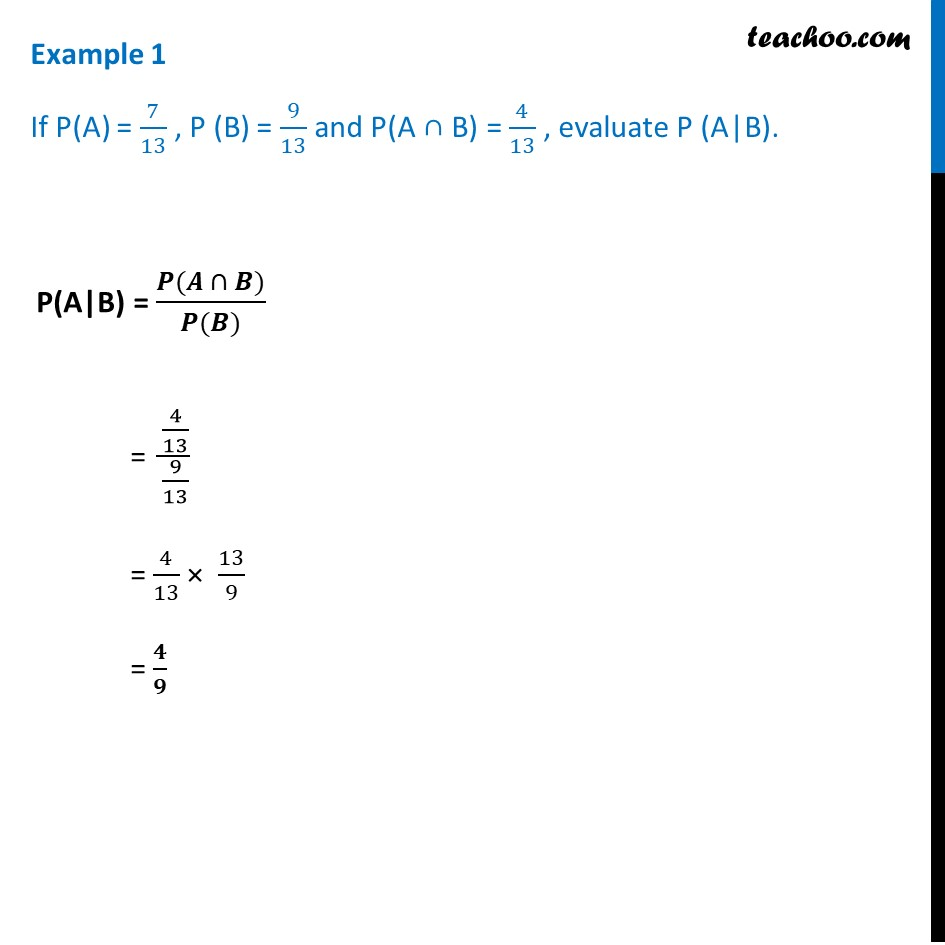Examples

Chapter 13 Class 12 Probability
Serial order wiseLearn in your speed, with individual attention - Teachoo Maths 1-on-1 Class

### Transcript

Example 1 If P(A) = 7/13 , P (B) = 9/13 and P(A ∩ B) = 4/13 , evaluate P (A|B). P(A|B) = (𝑷(𝑨 ∩ 𝑩))/(𝑷(𝑩)) = (4/13)/(9/13) = 4/13 × 13/9 = 𝟒/𝟗